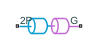# Interface (2P-G)

Two-phase fluid and gas network interface

Since R2021a

•Libraries:
Simscape / Fluids / Fluid Network Interfaces

## Description

The Interface (2P-G) block represents a flow connection between a two-phase fluid network and a gas network. Pressure, temperature, and mass flow rate are equal at the interface. The block does not transfer the fluid properties of each fluid network across the interface. To conserve fluid properties, ensure that the Gas Properties (G) block and the Two-Phase Fluid Properties (2P) block have corresponding settings.

The fluid at the 2P port must remain superheated. You can use the Two-phase fluid superheated vapor check parameter to choose what action the block performs if the fluid does not meet this condition.

### Mass and Momentum Balance

The block conserves mass such that

`${\stackrel{˙}{m}}_{2P}+{\stackrel{˙}{m}}_{G}=0,$`

where:

• ${\stackrel{˙}{m}}_{G}$ is the mass flow rate into port G.

• ${\stackrel{˙}{m}}_{2P}$ is the mass flow rate into port 2P.

The port pressures are equal:

`${P}_{G}={P}_{2P}.$`

### Energy Balance

The temperature is constant between the two fluid ports. The block calculates the energy balance over the interface as

`${\Phi }_{G}+{\Phi }_{2P}={\stackrel{˙}{m}}_{G}\left({h}_{G}+\frac{{w}_{G}^{2}}{2}\right)+{\stackrel{˙}{m}}_{2P}\left({h}_{2P}+\frac{{w}_{2P}^{2}}{2}\right),$`

where:

• ΦG is the energy flow rate into port G.

• Φ2P is the energy flow rate into port 2P.

• hG is the specific enthalpy at port G.

• h2P is the specific enthalpy at port 2P.

• vG is the specific volume at port G.

• v2P is the specific volume at port 2P.

• wG is the flow velocity at port G such that

`${w}_{G}=\frac{{\stackrel{˙}{m}}_{G}{v}_{G}}{S},$`

where S is the value of the Cross-sectional area at ports 2P and G parameter.

• w2P is the flow velocity at port 2P such that

`${w}_{2P}=\frac{{\stackrel{˙}{m}}_{2P}{v}_{2P}}{S}.$`

## Ports

### Conserving

expand all

Two-phase fluid conserving port associated with the two-phase fluid at the interface.

Gas conserving port associated with the gas at the interface.

## Parameters

expand all

Cross-sectional area of the two-phase fluid and gas ports.

Action to perform when the fluid at port 2P does not meet superheated conditions.

## Version History

Introduced in R2021a